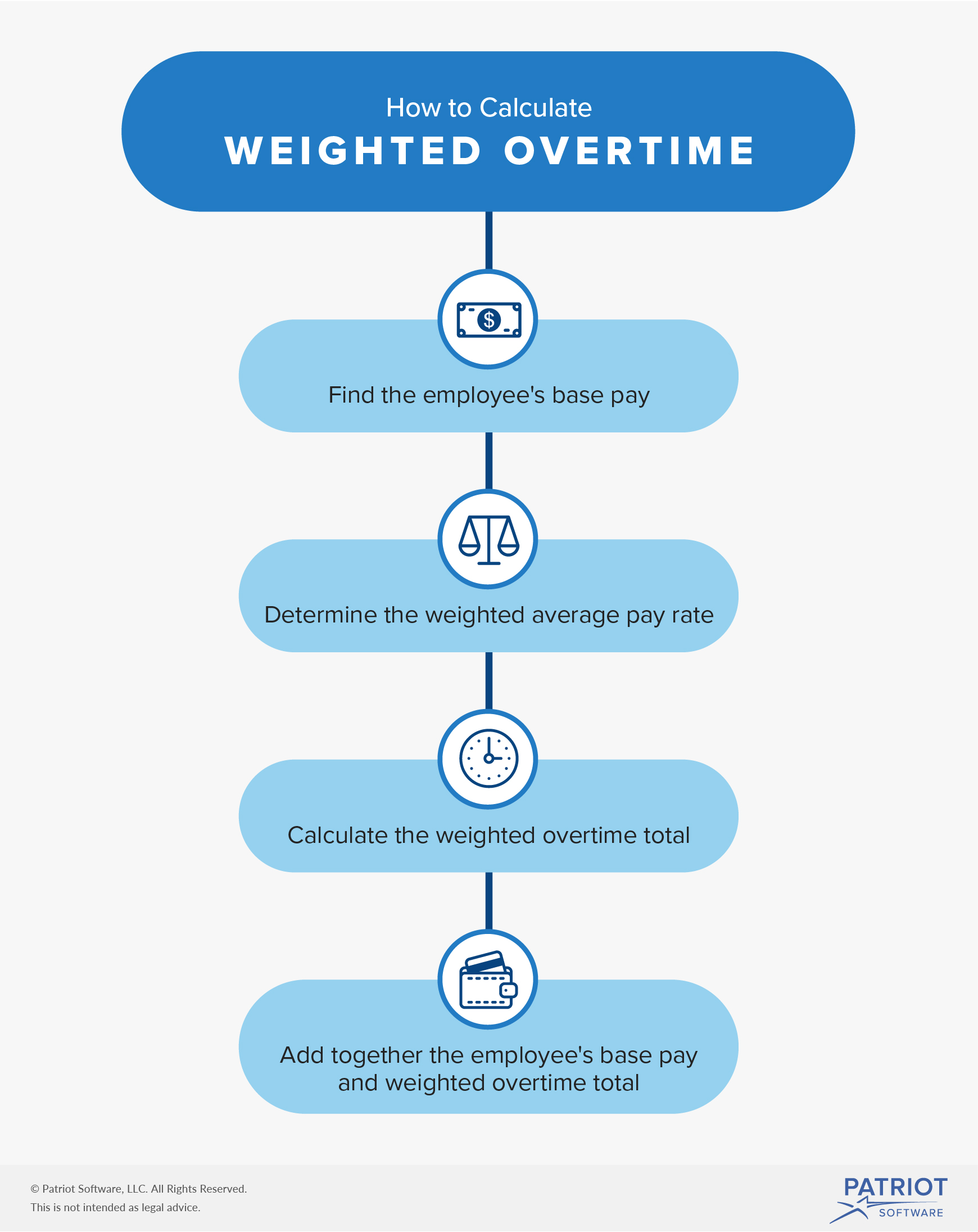Calculating Weighted Overtime for Employees With Multiple Pay Rates

# Calculating Weighted Overtime for an Employee With Multiple Pay Rates

Do you have an employee who has two or more positions in your business with different pay rates? Does that employee work overtime? If the answers to these questions are yes, you need to know about weighted overtime.

Weighted overtime is common in small businesses that pay multiple pay rates to an employee. Learn what overtime is, what weighted overtime is, and how to calculate weighted overtime.

## What is overtime?

Overtime is additional compensation you provide to nonexempt employees when they work more than 40 hours in a workweek. The federal overtime rate is time and one-half per hour worked beyond 40.

For example, if you have an employee who works 45 hours in a workweek, you need to pay them five hours of overtime. Multiply their hourly rate by the overtime rate of 1.5 to determine their overtime pay rate. Then, add the employee’s overtime wages to their regular earnings to get the total wages for the workweek.

The Fair Labor Standards Act (FLSA) governs overtime law. And, some states have overtime laws. You also need to know about FLSA weighted average overtime laws, explained below.

## What is weighted overtime?

Some employees work multiple positions within the same organization. Employers might pay an employee different pay rates for each job they work.

For example, you can have an employee who works as a waitress, hostess, and dishwasher in your business. You pay them \$5 per hour for waitressing, \$12 per hour for hostessing, and \$11 per hour for dishwashing.

If an employee who earns more than one hourly rate works overtime, how do you determine their overtime rate? Which hourly rate do you use to run payroll?

You can determine an employee’s overtime wages by finding the weighted average overtime. Weighted overtime, sometimes called blended overtime, is the average of an employee’s different pay rates. Then, you must use this weighted regular rate to find their overtime wages.

### How to calculate weighted overtime

Calculating weighted overtime is more extensive than calculating regular overtime wages, but it doesn’t have to give you a headache.

Blended overtime can be broken down into four steps: finding the employee’s base pay, determining the weighted average pay rate, calculating the blended overtime total, and finding the employee’s total compensation for the week.Use the following steps to calculate weighted overtime:

1. Find the employee’s base pay
• Multiply the hours the employee worked in each position by the hourly rates.
• Add together the total wages from both positions to get the total compensation.
2. Find the weighted average pay rate
• Divide the employee’s base pay by the number of hours worked to get the weighted average.
3. Find the weighted overtime total
• Multiply the weighted average by 0.5 to get the blended overtime rate.
• Multiply the weighted overtime rate by the number of overtime hours to get the total bonus overtime pay.
4. Find the total earnings
• Add together the employee’s base pay (Step 1) and their weighted overtime total (Step 3).

It’s easy to get overwhelmed by the formula steps. Use the following example to help you visualize the process.

#### Example

Let’s say you own a pizza shop. You pay an employee \$14 per hour when they make pizzas and \$10 per hour when they deliver. Your employee worked 30 hours making pizzas and 20 hours delivering them. Because the employee worked a total of 50 hours, you owe them overtime wages for 10 hours of work.

First, you need to find the employee’s base pay:

(30 hours X \$14) + (20 hours X \$10) = \$620

Next, find the employee’s weighted average pay rate by dividing their total compensation by the total number of hours worked, which is 50:

\$620 / 50 = \$12.40

Take the employee’s weighted average pay rate and multiply it by 0.5 to get their weighted overtime rate:

\$12.40 X 0.5 = \$6.20

Now, multiply the employee’s overtime rate by the number of overtime hours worked (10) to get their total overtime wages:

\$6.20 X 10 = \$62

Find the employee’s total compensation for the week by adding their base pay and weighted overtime wages:

\$620 + \$62 = \$682

The employee’s total compensation for the week is \$682.

### Alternative to weighted average overtime

You might be able to pay your employee an overtime rate based on the regular rate of the position in which they worked overtime.

According to the Department of Labor (DOL), you are only able to put away your weighted overtime calculator and use this method if you and your employee establish an agreement before they perform the work. And, the regular hourly rate used must be at least the minimum wage.

Be sure to check with your state’s wage and hour division to verify you can use the blended overtime alternative.

Let’s say an employee works as both a receptionist and a nail technician. The employee earns \$10 per hour as a receptionist and \$17 per hour as a nail technician. One week, the employee works four hours of overtime performing receptionist duties. And, the employee works two hours of overtime performing nail technician duties. You would compute the employee’s four hours of overtime as a receptionist using their hourly pay rate of \$10. You would also compute their two hours of overtime as a nail technician using their regular rate of \$17 per hour.

Ready to simplify overtime calculations? Patriot Software’s online payroll software makes it easy to record, calculate, and pay your employees’ regular and overtime hours. Our easy-to-use software is designed for small business owners. Get your free trial now!